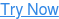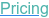#### SOFTWARE#### KNOWLEDGE BASE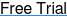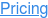# Sample Size for Statistical Intervals

June 7, 2022

Statistical intervals are a vital tool for understanding the uncertainty for an estimate.

Confidence intervals are commonly used with sample size determination methods based on a target level of precision for many scenarios. However, sample size methods also exist for other intervals such as prediction and tolerance intervals as well as Bayesian approaches such as credible intervals.

## Sample Size for Statistical IntervalsCalculations for Confidence, Prediction and Credible Intervals### In this free webinar, you will learn how to overcome the challenges associated with:

• Introduction to Statistical Intervals and Sample Size
• Sample Size for Confidence Intervals
• Sample Size for Prediction and Tolerance Intervals
• Sample Size for Bayesian Credible Intervals

Statistical intervals are an essential tool for understanding the uncertainty in our estimates from statistical tests and models. Sample size determination methods can help a trialist understand how many subjects are needed to achieve a required level of precision in the intervals of most interest.

Confidence intervals are the most widely used type of statistical interval which gives the expected uncertainty for a parameter under repeated sampling. Sample size methods for confidence intervals are developed for a wide variety of endpoints, regression models and study designs. These sample size methods typically target a specific level of precision in the estimate.

However, sample size determination also exists for other less common interval types. Examples include Prediction Intervals, Tolerance Intervals and Bayesian Credible Intervals. We explore each of these intervals, how they compare to confidence intervals and what is the target of interest in their respective sample size methods.

In this webinar, we introduce the variety of statistical intervals available and describe methods to calculate the required sample size for confidence, prediction, tolerance and credible intervals.

# Play the video below to watchthe complete recording of this webinar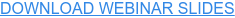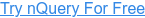Looking for more resources?

## You May Also Like

These Stories on Guide to Sample Size